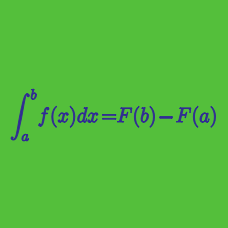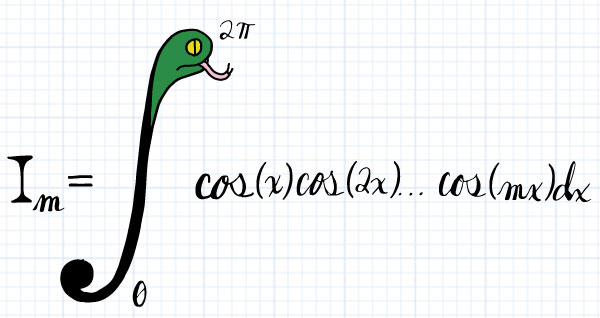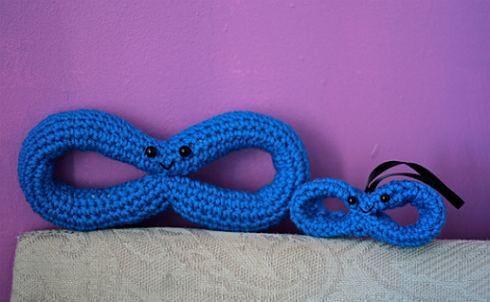Calculus

# Definite Integrals: Level 3 Challenges

$\displaystyle \int_{0}^{20} \Bigl(\lfloor x \rfloor \{x\} \Bigr) \ dx = \ ?$

Details and assumptions:

• Every $x\in \mathbb{R}$ can be written as $x=\lfloor x \rfloor + \{x\}$.

• $\lfloor x \rfloor$ denotes greatest integer less than or equal to $x$.

• $\{x\}$ is the fractional part of $x$.Let $I_m = \displaystyle \int_0^{2\pi} \cos(x) \cos(2x) \dots \cos(mx) dx$. What is the sum of all integers $100 \leq m \leq 110$ such that $I_m \neq 0$?$\displaystyle{ S }_{ N }=\sum _{k=1}^{N}\frac1k$

Let $S_N$ satisfy the equation above. What is the value of $\displaystyle \lim _{ n\rightarrow \infty }{ \left( { S }_{ 2n }-{ S }_{ n } \right) }$?

Give your answer to three decimal places.

$f(x) = \begin{cases}{1-|x|}, && {|x|\>\le\>1} \\ {|x|-1,} && {|x|>1}\end{cases}$

$g(x) = f(x-1)+f(x+1)$.

Given the two functions above, what is the value of $\displaystyle \int _{ -3 }^{ 5 }{ g(x) \mathrm{d}x }$?

$\large \int_0^{\pi /2} ( \sin^{2014}x - \cos^{2014}x ) \,dx = \, ?$

×

Problem Loading...

Note Loading...

Set Loading...